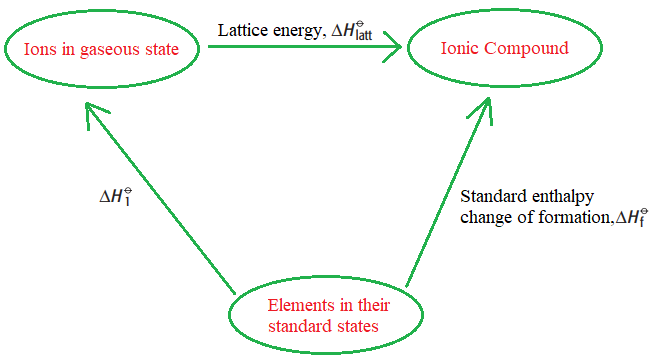In a solid ionic crystal lattice, the ions are bonded by well-built ionic bonds between them. These forces are only completely smashed when the ions are in a gaseous state.

Lattice energy is the enthalpy change when one mole of a solid ionic lattice is formed from its scattered gaseous ions. Lattice energy is always negative. This is because energy is always released when bonds are formed.

Defining lattice energy

Lattice energy is an estimate of the bond stability in ionic compounds. It is defined as the heat of formation for ions of opposite charge in the gas phase to combine into an ionic solid.

Outline

## Enthalpy change of atomization

The Enthalpy change of atomization is the enthalpy change when one mole of a gaseous atom is formed from its elemental state under standard conditions.

Standard conditions are the temperature of 298 K and a pressure of 101 kPa

Example

The standard enthalpy change of atomization of lithium is

Li(s) to Li(g) ΔHθ at = +161 kJmol–1

The standard enthalpy change of atomisation of chlorine is

1/ 2Cl2(g) to Cl(g) ΔH θ at = +122 kJmol–1

Values of ΔH —O at are always positive (endothermic) because energy must be supplied to break the bonds holding the atoms in the element together.

## Born Haber cycle

It is a type of enthlapy cycle used to calculate the lattice energy.Before understanding the Born-haber cycle some important concepts are Inoization energy, eclectron affinity,dissociation energy,sublimation energy,heat of formation,and Hess’s Law. The Born-Haber Cycle can be applied to determine all of these.

Inoization Energy

Inoizaton energy is the energy required to remove an electron from a neutral atom or an ion. Generally it increases across the period from left to right. It alwyas have positive value.

Electron Affinity

Electron affinity is the energy released when an electron is added to a neutral atom or an ion. Generally, it increases across the period in the periodic talbel from left to right and decreases in group from to bottom.

Dissociation energy.

Bond dissociation energy is the amount of energy requried per mole when a particular chemical bond is broken in a gaseous state of matter. It depends on the nature of atoms.

Sublimation energy

Sublimation energy is the amount of energy required to change the phase from solid to gas, by skipping the liquid phase. It has a positive value because it is an input energy which is given to the system.

Heat of formation

The change in energy (either absoberb or evolve) when a compound is formed from its elements.Heat of formation, also called standard heat of formation, enthalpy of formation, or standard enthalpy of formatio.This may be positive or negative.

Hess’s Law

Hess’s Law states that the energy change in an overall chemical reaction is equal to the sum of the energy changes in the individual reactions comprising it. In other words, the enthalpy change of a chemical reaction (the heat of reaction at constant pressure) does not depend on the pathway between the initial and final states. The law is a variation of the first law of thermodynamics and conservation of energy.

## Components of the Bron Haber cycle## Born Haber Cycle Examples

Born Haber cycle of sodium chloride NaCl

Na(S) + 1/2 Cl(g) → NaCl(S) ΔHf0 = -411 kJ/mol

The formation of ionic solid is not a single step it gose through multiple step

1st Step

Solid sodium atom sublimes to gaseous atom by absorbing heat energy (∆Hsub)

Na (s) → Na (g)

Sublimation energy ΔHsub = + 107kJ/mol

2nd Step

Gaseous sodium atom absorbs the ionization energy to release one electron and forms a gaseous sodium ion.

Na(g) → Na+(g) + 1e ,

Ionization energy ∆HIE = +502kJ/mol

3rd Step

Now chlorine breaks into two individual atoms by absorbing bond energy, such that each chlorine atom absorbs half of the bond energy of the chlorine molecule.

Cl2(g) → 2Cl(g)

Bond dissociation energy of chlorine = 1/2 ∆Hdiss = 242/2 =+121 KJ/mol

4th Step

Chlorine atom accepts an electron to form chloride ion and releases energy equivalent to electron affinity.

Cl(g) + 1e– → Cl(g)

Electron affinity = ∆HEA = -355kJ/mol

5th Step

## Enthalpy change of solutions

Example

As an example, the lattice energy of sodium chloride, NaCl, is the energy released when gaseous Na+ and Cl ions come together to form a lattice of alternating ions in the NaCl crystal.

Na+(g) + Cl (g) ——-> NaCl(S) Δ H = -787.3 KJ/mol

Factors affecting the lattice Energy

There are two factors that affect the lattice energy

• Charge on the ions.
• The radius of the ions.

Charge on the ions.

The more the charge on the ion, the more the lattice energy.

This is due to ions of greater charge having stronger attraction towards each other. Hence high energy is released when bonds are formed between them.

For Example,

Magnesium oxide, MgO has more lattice energy than sodium chloride. This is because Mg²⁺ and O²⁻ have greater charge than Na⁺ and Cl⁻ ions.

The larger the ionic radius, the lesser the lattice energy.

This is due to the attraction between ions getting weaker as the distance between them increases. Hence less energy is released when bonds are formed between them.

For Example,

The lattice energy of sodium halides gets lower when you move from sodium fluoride to sodium iodide. This is because as the size of the halide ion increases, the distance between sodium and halide ions increases.